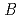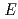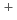For the nuclei where axially asymmetric or reflection-asymmetric shapes are expected, we calculated the nuclear charge radii by using the Finite Range Droplet Model. As a result, we found the agreement between the experimental and theoretical radii becomes better when these asymmetries are taken into account in the model calculation. In addition, we developed a method to empirically calculate the charge radii of even-even nuclei. This method is based on the Finite Range Liquid Drop Model, and use the experimental(2) value or the excitation energy of the first excited 2state as input parameters.